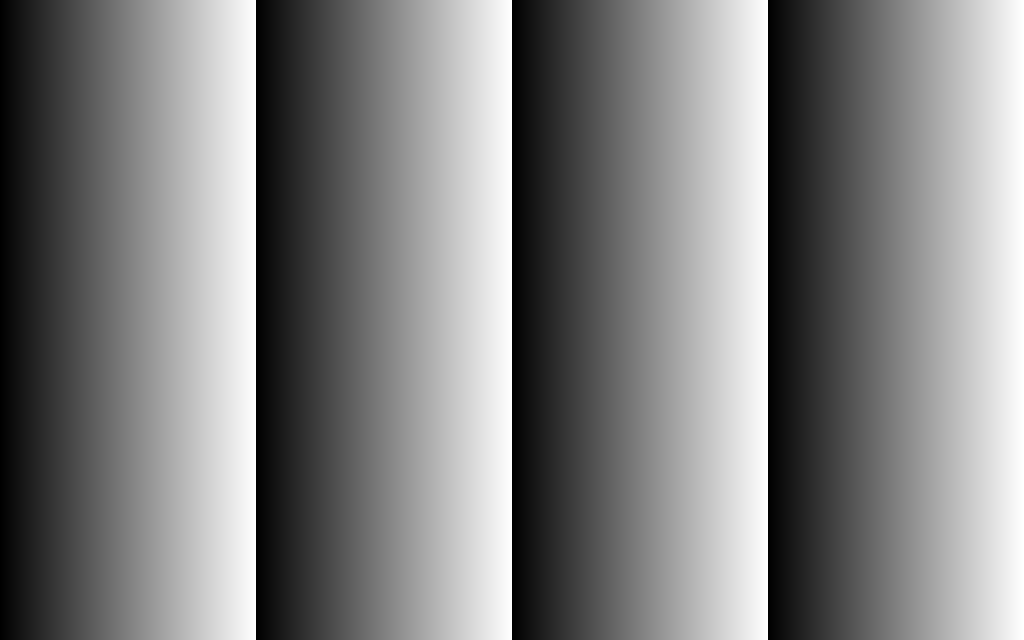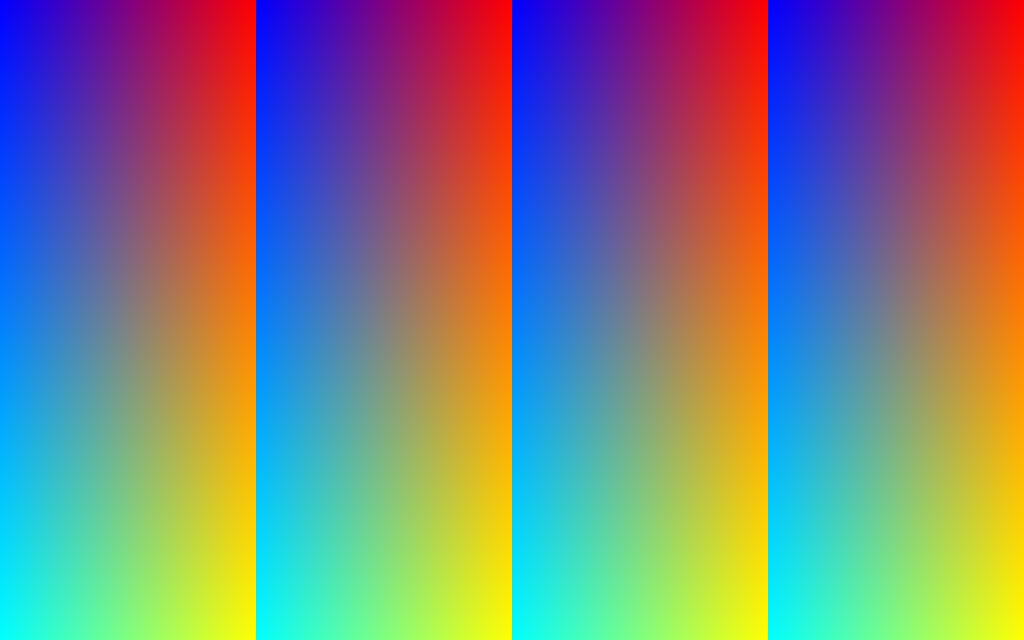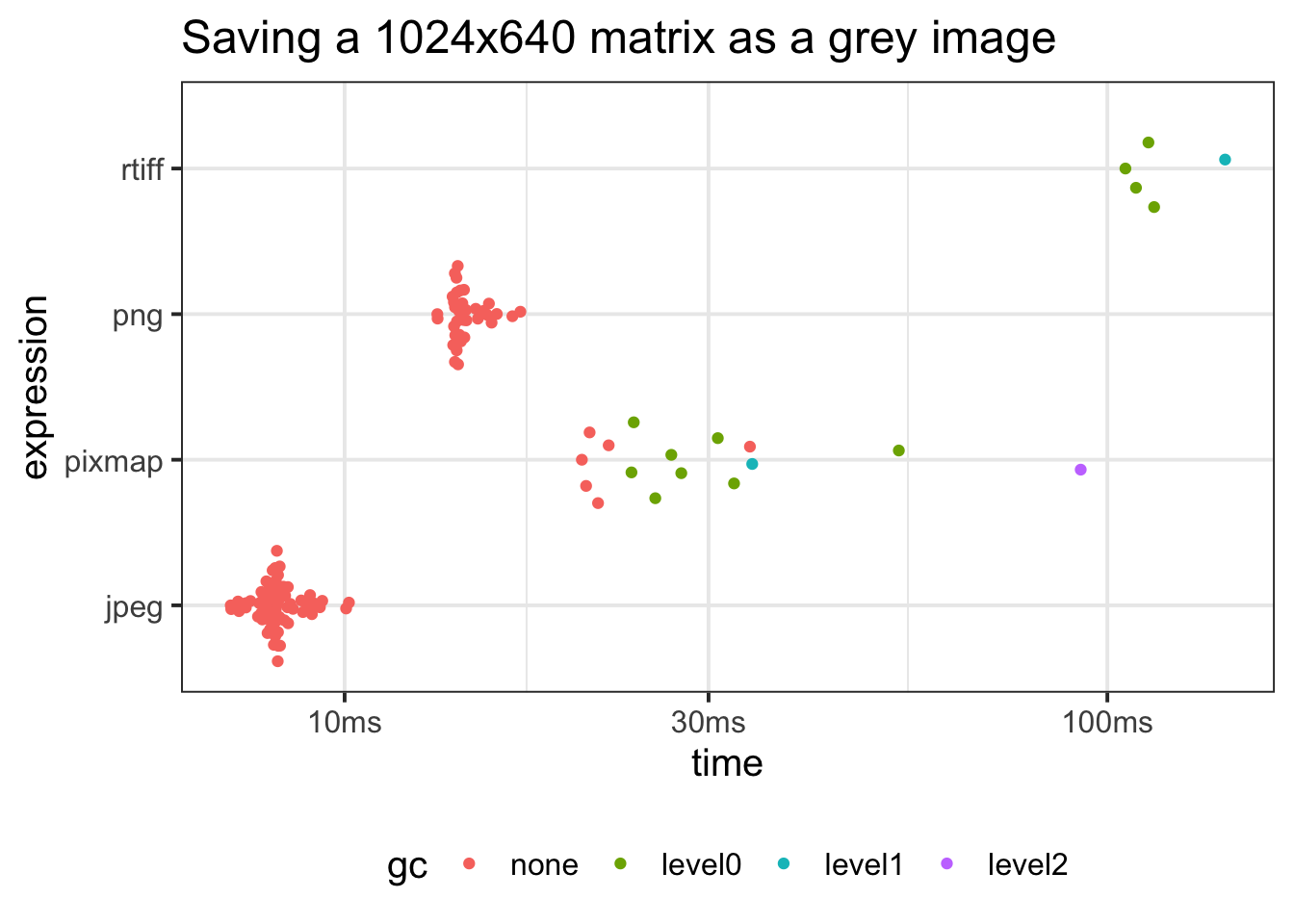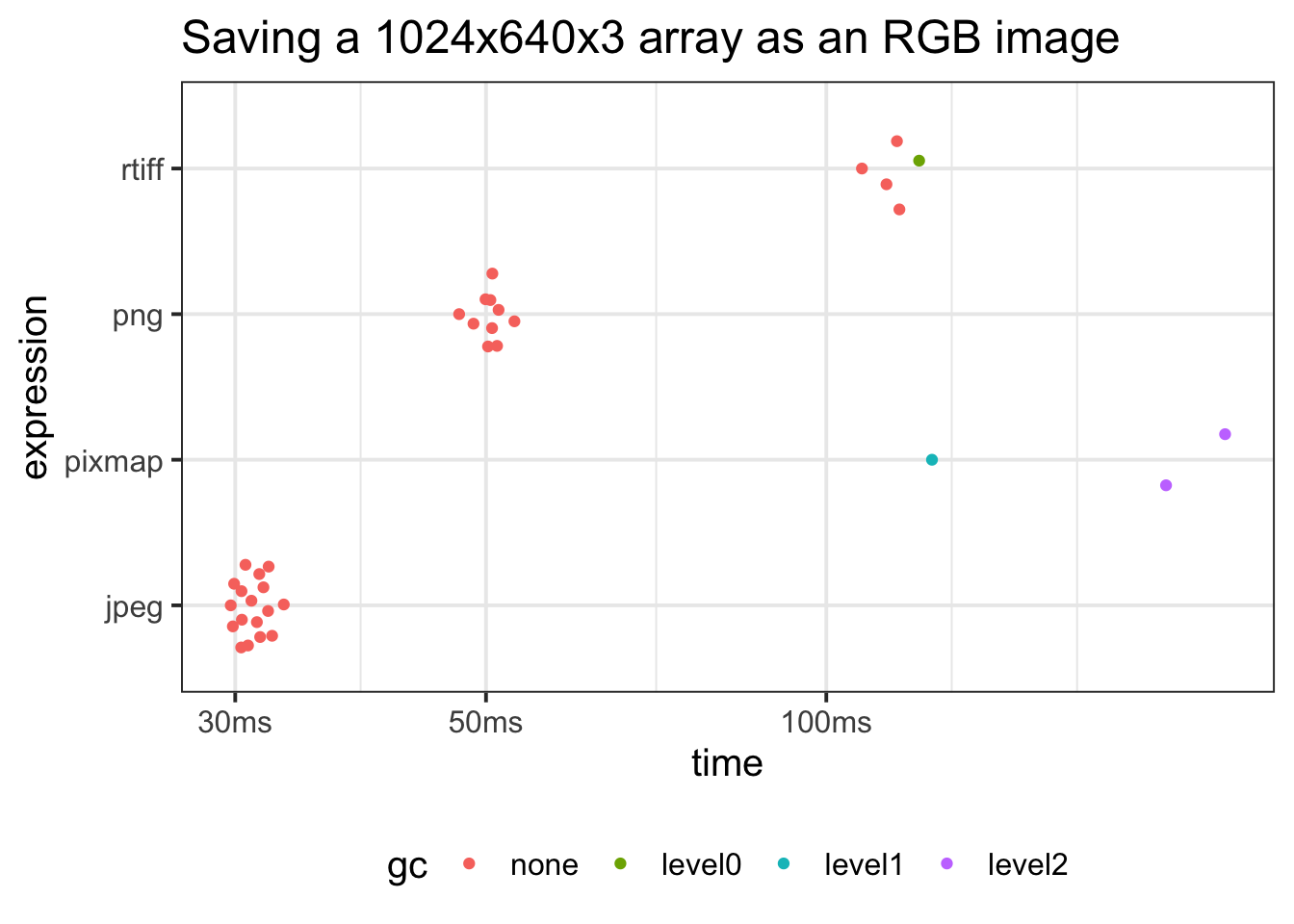# Outputting data to images

## Problem: Saving lots of R matrices and arrays to image files

A current project generates 100s (even 1000s) of matrices which I’d like to view outside of R i.e. I’d like to save all these matrices as image files and then process them with other tools.

This post benchmarks some ways of saving a matrix or an array as an image file.

Notes:

• My machine has an SSD.
• Timing will fluctuate due to all sorts of disk related IO factors - I’m not too concerned at this stage, I just want a rough idea on ranking by speed.
• Compressed data may be actually written to disk faster (less to write), but it takes time to actually compress the data (more work to do). So there’s probably a sweet spot in there.
• I don’t want to use a lossy format, but I’ve included `jpeg` output for comparison, as the jpeg library itself has been optimized over decades and is very, very fast.
• All my data is in matrix objects - if a library needs to convert to some particular data structure before output, then that’s part of its benchmark time.

## Data Setup

``````ncol    <- 1024
nrow    <- 640
int_vec <- rep.int(seq(ncol) - 1, nrow) %% 256L
int_mat <- matrix(int_vec, nrow = nrow, ncol = ncol, byrow = TRUE)
dbl_mat <- int_mat/255

r       <- dbl_mat
g       <- matrix(rep(seq(0, 255, length.out = nrow)/255, each = ncol), nrow, ncol, byrow = TRUE)
b       <- dbl_mat[, rev(seq(ncol(dbl_mat)))  ]

dbl_arr <- array(c(r, g, b), dim = c(nrow, ncol, 3))``````

#### dbl_mat#### dbl_arr## Comparing packages

• png
• jpeg
• pixmap - Requires that arrays and matrices are first converted to pixmap objects.
• rtiff - Requires that arrays are first converted to pixmap objects.

## Writing a matrix as a grey image

``````#~~~~~~~~~~~~~~~~~~~~~~~~~~~~~~~~~~~~~~~~~~~~~~~~~~~~~~~~~~~~~~~~~~~~~~~~~~~
# Integer matrix saved to image
#~~~~~~~~~~~~~~~~~~~~~~~~~~~~~~~~~~~~~~~~~~~~~~~~~~~~~~~~~~~~~~~~~~~~~~~~~~~
tmp <- tempfile()

res <- bench::mark(
png    = png::writePNG                       (dbl_mat, tmp),
jpeg   = jpeg::writeJPEG                     (dbl_mat, tmp),
pixmap = pixmap::write.pnm(pixmap::pixmapGrey(dbl_mat),tmp),
rtiff  = rtiff::writeTiff(                    dbl_mat, tmp),
check = FALSE
)``````
Table 1: Saving a 1024x640 matrix as a grey image
expression min median itr/sec mem_alloc
png::writePNG(dbl_mat, tmp) 13.23ms 14.14ms 69 670.8KB
jpeg::writeJPEG(dbl_mat, tmp) 7.09ms 8.15ms 122 661.2KB
pixmap::write.pnm(pixmap::pixmapGrey(dbl_mat), tmp) 20.46ms 26.18ms 31 32.3MB
rtiff::writeTiff(dbl_mat, tmp) 105.61ms 113.2ms 9 94.2MB
``Loading required namespace: tidyr``## Writing an array as an RGB image

``````#~~~~~~~~~~~~~~~~~~~~~~~~~~~~~~~~~~~~~~~~~~~~~~~~~~~~~~~~~~~~~~~~~~~~~~~~~~~
# Write an RGB integer array to image
#~~~~~~~~~~~~~~~~~~~~~~~~~~~~~~~~~~~~~~~~~~~~~~~~~~~~~~~~~~~~~~~~~~~~~~~~~~~
pm <- pixmap::pixmapRGB(dbl_arr)

res <- bench::mark(
png            = png::writePNG                      (dbl_arr,  tmp),
jpeg           = jpeg::writeJPEG                    (dbl_arr,  tmp),
pixmap         = pixmap::write.pnm(pixmap::pixmapRGB(dbl_arr), tmp),
rtiff          = rtiff::writeTiff (pixmap::pixmapRGB(dbl_arr), tmp),
check = FALSE
)``````
Table 2: Saving a 1024x640x3 array as an RGB image
expression min median itr/sec mem_alloc
png::writePNG(dbl_arr, tmp) 47.3ms 50.5ms 20 1.88MB
jpeg::writeJPEG(dbl_arr, tmp) 29.7ms 31ms 32 1.88MB
pixmap::write.pnm(pixmap::pixmapRGB(dbl_arr), tmp) 124ms 199.8ms 5 135.04MB
rtiff::writeTiff(pixmap::pixmapRGB(dbl_arr), tmp) 107.5ms 115.5ms 9 60.02MB## Summary

• `png` output is fast.
• `jpeg` output is very, very fast.
• `pixmap` and `rtiff` are both slow and allocate ~2 orders of magnitude more memory than `jpeg` or `png`.